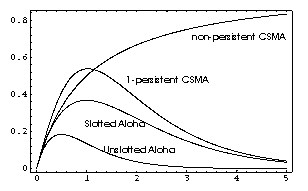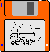### JPL's Wireless Communication Reference Website

#### Chapter: Data Networks Section: Random Access# Throughput of Random Access Schemes

An important measure of performance is the throughput.

• The offered or attempted traffic, denoted as G, is the number of transmission attempts that is made on the channel, per unit of time.
In non-persistent CSMA, attempts made when the channel is busy are inhibited. These attempts contribute to the offered traffic.
• Throughput, denoted as S, is the average fraction of time that the radio channel successfully carries packets.
In slotted systems, the slot length is taken as the unit of time.

In virtually all protocols (including ALOHA, CSMA, ISMA), S is approximately equal to G for small G, i.e., if the channel is lightly loaded. At larger traffic loads (larger G), conflicts, called "collisions" occurs, so S < GFigure: Throughput S [in packets per unit of time] versus attempted traffic G for slotted ALOHA, unslotted ALOHA, non-persistent CSMA and 1-persistent CSMA

### Throughput Analysis

 Algorithm Throughput S S for G = Average number of attempts G/S Slotted ALOHA S = G exp[-G] S = G/S= Unslotted ALOHA S = G exp[-2G] S = G/S= Nonpersistent CSMA and ISMA S = G /(1+G) S = G/S= 1-persistent CSMA and ISMA S = (G + G2) / (1 + G * exp[G]) S = G/S=

Non-persistent CSMA / ISMA is the only curve for which S does not reduce to zero for large G. If terminals can indeed infinitely fast sense the channel, as is assumed here, collisions can be avoided completely.

Note that the average number of (re-) transmission attemps only equals G/S in a stable system. Stability is problematic is retransmission waiting times are short in a network with many terminals.

###Mathematica Source Code

```Plot[{G Exp[-G], G Exp[-2 G], G /(1+G),
(G + G*G) / (1 + G * Exp[G])}, {G, 0, 5 },
Frame -> True]
```

### Performance over wireless channels

In a wireless channel performance differs substantially from the performance over cable Local area Network.
• Packets may be lost due to fading even if no collision occur
• If collisions occur not all packet involved are lost. Some may nonetheless be detected successfully, particularly if these are received with strong power. This effect is called capture.
The following figures compare various random access methods for the following set of parameters and assumptions:
• Average message arrival and retransmission rate: 1 packet per unit of time.
• Terminals quasi-uniformly distributed over cell, with cell boundary approximately at unity distance.Figure: Probability that an access attempt is successful versus the distance between terminal and base station. orange: slotted ALOHA blue: non-persistent ISMA, with signalling delay d. The attempt may fail if the terminal is inhibited. green 1-persistent ISMAFigure: Throughput (in packet per unit of time) versus attempted traffic.